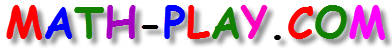#Play this cool Math Magician Addition Game and make 20 bunnies disappear by quickly matching addition problems with the correct answers. Drag and drop the bunny into the correct basket to make it disappear and score points to win.

This interactive game provides first grade students with an engaging way to develop their addition skills. The game can be played on computers, iPads, and other tablets. You do not need to install an app to play this game on the iPad.

The game is aligned to the following Common Core math standards:

CC1.OA.1
Use addition and subtraction within 20 to solve word problems involving situations of adding to, taking from, putting together, taking apart,
and comparing, with unknowns in all positions, e.g., by using objects, drawings, and equations with a symbol for the unknown number to
represent the problem.

CC1.OA.6
6. Add and subtract within 20, demonstrating fluency for addition and subtraction within 10. Use strategies such as counting on; making ten
(e.g., 8 + 6 = 8 + 2 + 4 = 10 + 4 = 14); decomposing a number leading to a ten (e.g., 13 – 4 = 13 – 3 – 1 = 10 – 1 = 9); using the relationship between addition and subtraction (e.g., knowing that 8 + 4 = 12, one knows 12 – 8 = 4); and creating equivalent but easier or known sums (e.g., adding 6 + 7 by creating the known equivalent 6 + 6 + 1 = 12 + 1 = 13).